# How to group dates by number of days in Excel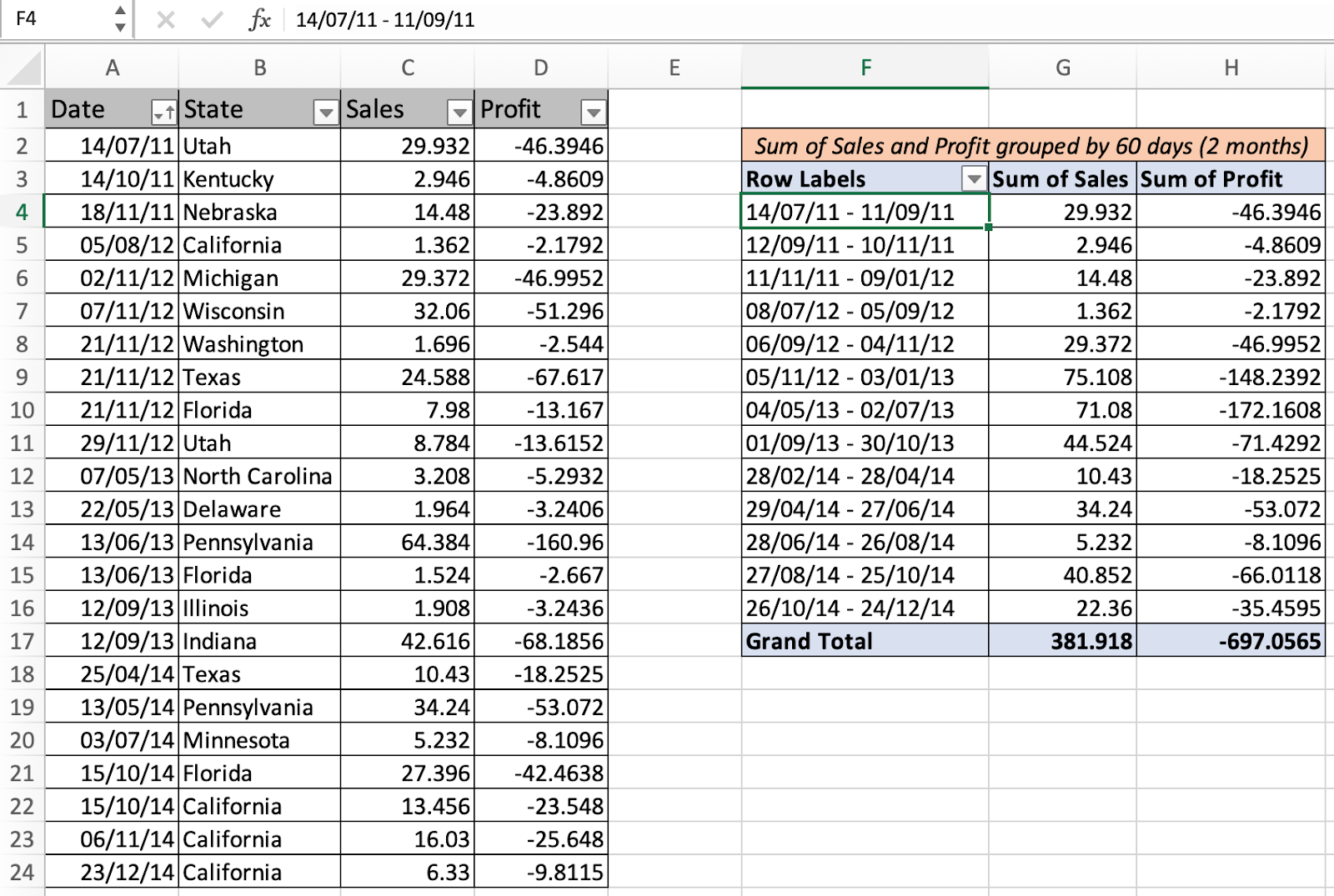In this article, we will learn How to group dates by the number of days in Excel.

Scenario:

Problem is when we have a set of date fields in the pivot table. And the user wants to group the date field by some number of days inplace of years or discrete dates. For example grouping the import and export of products in each 60 days in Excel. Or grouping employee data by months or weeks in Excel. For problems like these we use pivot tables and grouping options. There is one other way for the users who use aggregate function. Let's understand these two processes one by one.

Group in pivot table in Excel

Select data > Insert > Pivot table > (Insert a new pivot table) > Go to pivot table add date field to rows > Right click date value > Group… > Choose months or days > Click Ok to confirm

Alternate way with aggregate function

Add starting date and increment cell at each interval of 60. Use the aggregate excel formula having multiple criteria.

Example :

All of these might be confusing to understand. Let's understand how to use the function using an example. Here we have a sample sales and profit data by states. We need to group the data by 60 days (approx 2 months).Sort the data by date field. Select the table and insert a pivot table for data. Add date to rows and sales and profit to values.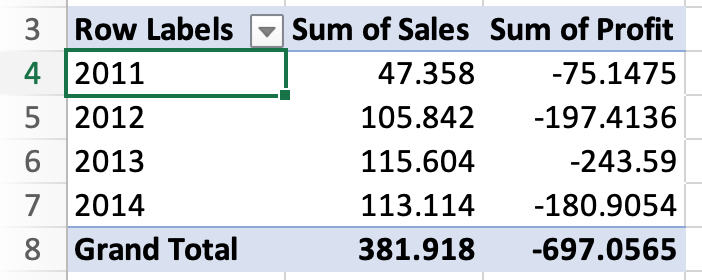As you can see the date field is in year format. But we want to group by 60 days. So right click date value > Select Group…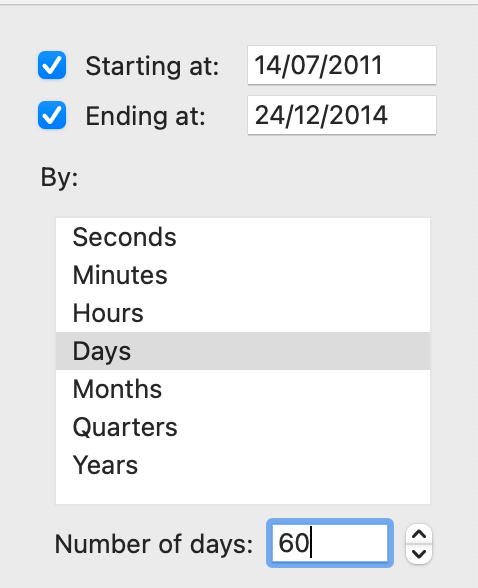Click Ok.

Date is grouped by 60 day interval along with sales and profit value.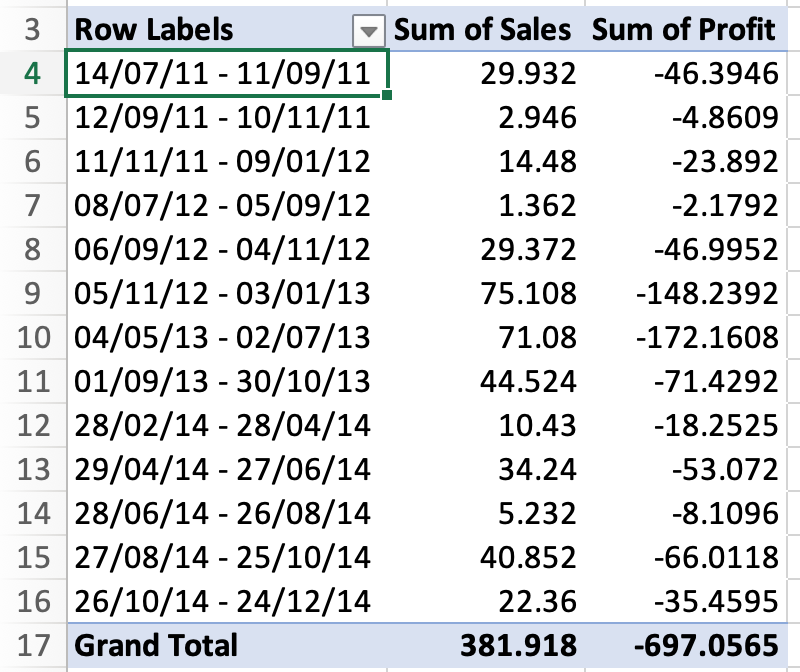This is the easiest way to group date by months. But if you need the above data using sumifs formula it will be more time consuming but it's convenient to use functions efficiently.

Alternate way using SUMIFS formula

For the same data make a new table naming the starting date and add 59 days to the starting date as shown below.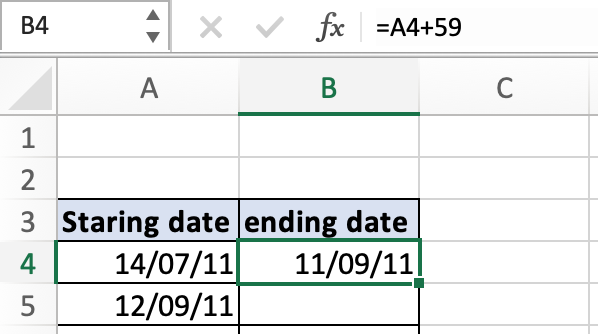And in the A5 cell use =B4+1 to get the next date. Now copy and paste the B4 cell formula till it gets the end date. And copy paste the A5 cell formula till the end date range.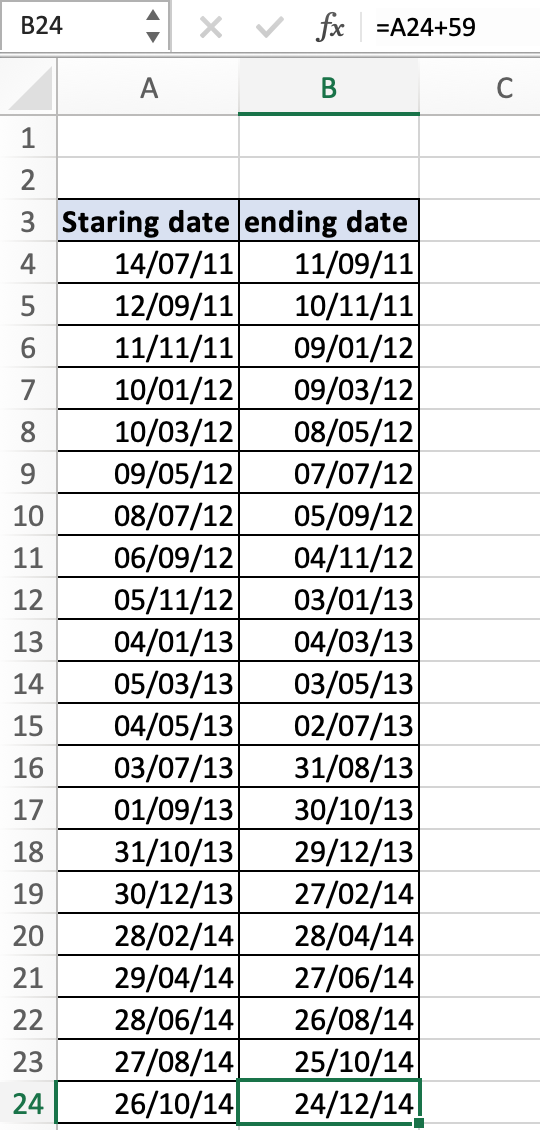Now use the sumifs formula

=SUMIFS( sum range, date column from table , ">=" & A4 , date column from table , "<=" & B4 )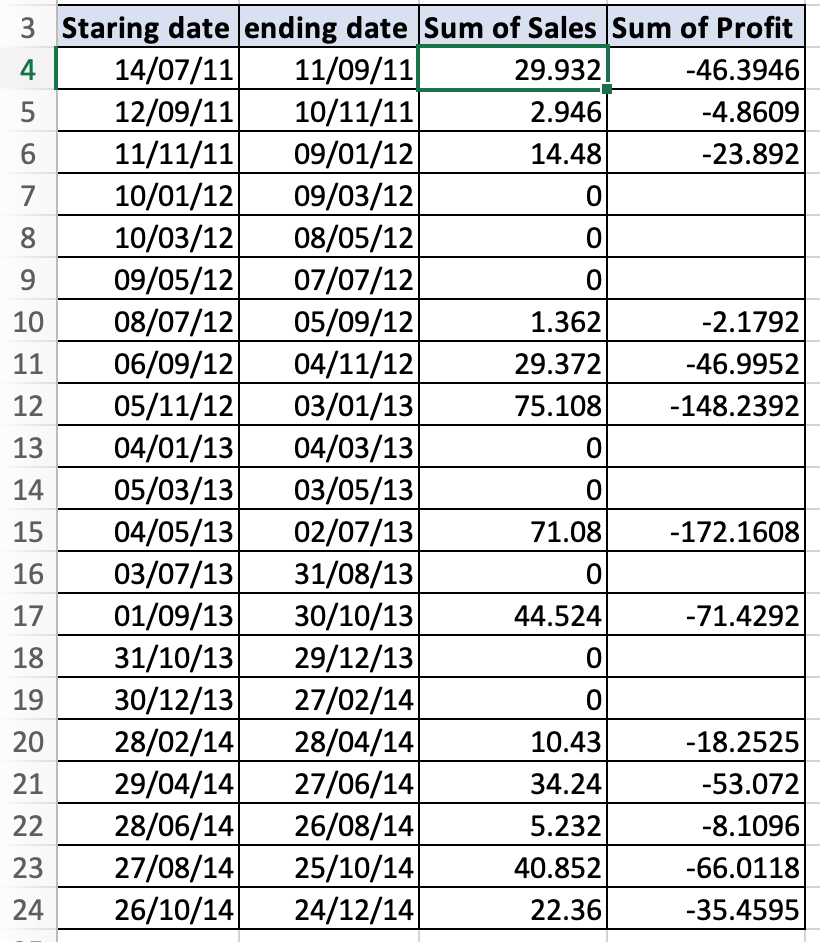Filter the blank or 0 value cell to get the same field we obtained using the pivot table.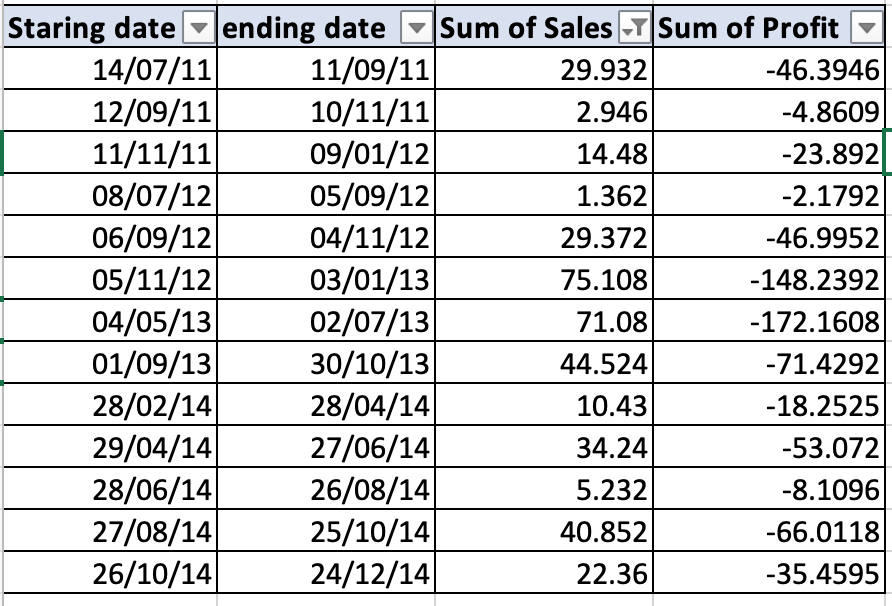Whereas pivot table provides you with different aggregate functions like average, count, sum, max, min, var, stdev, But excel functions AVERAGEIFS, COUNTIFS, SUMIFS, MAXIFS and MINIFS to choose from. MAXIFS and MINIFS are update functions above excel 2016 or new Excel 365.

Here are all the observational notes using the explained method in Excel
Notes :

1. Prefer or use the pivot table method, it's easy and has many functions to choose from.
2. Excel stores dates and time as numbers and is used in calculation by the function. So it’s recommended to use dates as cell reference or using the DATE function instead of giving direct argument to the function. Check the validation of date values, or else the function returns #VALUE! Error.
3. Use quotes with sumifs formula for ">=" and "<=".
4. MAXIFS and MINIFS new and updated version of MIN and MAX with criteria. Or use the array formula with MAX and MIN in place for MINIFS and MAXIFS older versions of excel.

Hope this article about How to group dates by number of days in Excel is explanatory. Find more articles on calculating date values and related Excel formulas here. If you liked our blogs, share it with your friends on Facebook. And also you can follow us on Twitter and Facebook. We would love to hear from you, do let us know how we can improve, complement or innovate our work and make it better for you. Write to us at info@exceltip.com.

Related Articles :

Excel Pivot Tables : Pivot tables are one of the most powerful tools and one who knows all the features of pivot tables can increase his productivity exponentially. In this article we will learn all about pivot tables in detail.

Count holidays between dates in Excel : count non working days between the two given dates using the DATEDIF and NETWORKDAYS function in Excel.

Conditional Formatting for Pivot Table : Conditional formatting in pivot tables is the same as the conditional formatting on normal data. But you need to be careful while conditional formatting pivot tables as the data changes dynamically.

How to Convert date to text in Excel : In this article we learned how to convert text into date, but how do you convert an excel date into text. To convert an excel date into text we have a few techniques.

Extract days between dates ignoring years in Excel : count the days between the two dates using different functions and mathematical operation in Excel.

Count Birth Dates By Month in Excel : count the number of dates lying in a particular month using the SUMPRODUCT and MONTH function in Excel.

How to use the NETWORKDAYS function in Excel : Returns the working days between the two given dates using the NETWORKDAYS function.

Popular Articles :

How to use the IF Function in Excel : The IF statement in Excel checks the condition and returns a specific value if the condition is TRUE or returns another specific value if FALSE.

How to use the VLOOKUP Function in Excel : This is one of the most used and popular functions of excel that is used to lookup value from different ranges and sheets.

How to use the SUMIF Function in Excel : This is another dashboard essential function. This helps you sum up values on specific conditions.

How to use the COUNTIF Function in Excel : Count values with conditions using this amazing function. You don't need to filter your data to count specific values. Countif function is essential to prepare your dashboard.

1.